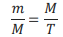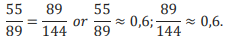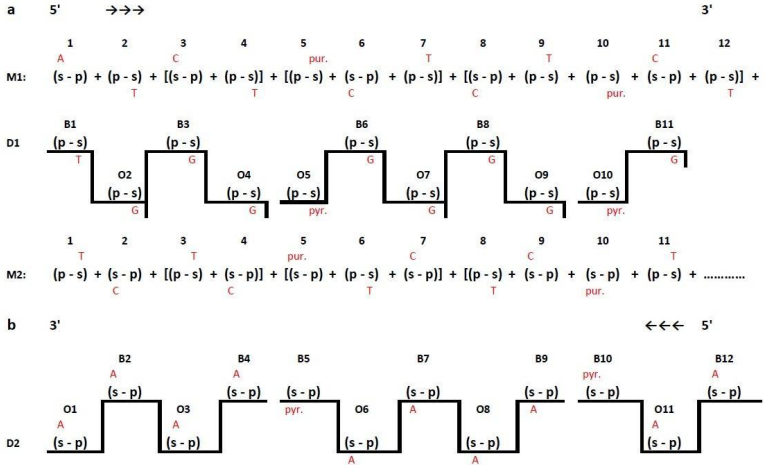# DNA STRUCTURE, REPLICATION AND GENOME VARIABILITY CORRELATION

Research article
Issue: № 1 (1), 2016
Published:
15.09.2016

DNA STRUCTURE, REPLICATION AND GENOME VARIABILITY CORRELATION

Conflict of Interest

None declared.

Shabalkin I.P.1, *, Gudkova M.V.2, Stukalov Yu.V.3, Grigorieva E.Yu.4, Cherepanov A.A.5, Shabalkin P.I.6

1,2,3,4,5,6Blokhin Cancer Research Center, Kashirskoe sh.24, Moscow

*To whom correspondence should be addressed.

Associate Editor: Giancarlo Castellano

Received on 23 May 2016; revised on 03 August 2016; accepted on 15 September 2016

Abstract

The study of special features of a new molecular DNA structure synthesis based on the fact that monomers transpositions can occur in the backbone of polymer chains according to the mathematical law known as a Fibonacci numerical series and «the Golden ratio» was performed. The example of the formation of a new DNA model demonstrates that there are dimers of three types in DNA structure:

1. Dimers with phosphodiester bond P-O-C, [(s-p) + (s-p)]; [(p-s)+(p-s)];

2. Dimers with phosphatic bond P-O-P, [(s-p) + (p-s)];

3. Dimers with glycosidic bond C-O-C, [(p-s)+(s-p)].

Dimers of the [(s-p) + (p-s)] type are of special importance for the process of replication. For example, if an enzyme catalyst (DNA polymerase) interacts in the backbone of matrix thread with a dimer of [(s-p) + (p-s)] type during the replication process it leads to a thread break. The growth of the daughter thread does not occur until the enzyme catalyst finds the transition point to another matrix thread of DNA, which contains in its backbone monomers similar to those, necessary for the activity of the given DNA polymerase. Thus, during the process of replication the genetic material is redistributed in the cell, and each daughter thread gets the information about genes belonging to both matrix threads of DNA molecule.

This pattern of cell genome changing may manifest itself in phenotype or genotype of the body in different ways.

Supplementary information: Supplementary data are available at Journal of Bioinformatics and Genomics online.

Keywords: DNA structure, replication, genome variability, correlation.

Contact: shabalkin.i.p@mail.ru

It is known that DNA molecule serves as the genetic basis for the genome functioning. According to Watson-Crick DNA model the backbone of macromolecule is formed by monomers linked with each other by repeated structures composed from saccharide-deoxyribose and phosphoric acid residue-phosphatic group (PO4).It is assumed that these repeated units in the polymer chain backbones should follow strictly one after another in the same position set by the model.

This approach to the DNA model has prevailed in research articles for more than 50 years. However, by now this point of view doesn’t seem to be convincing. M. Singer and P. Berg (Singer, 1988) have supposed that opinion, existed until recently, that DNA B-form which is a perfect double helix with stable geometrical parameters independent of the sequence of nucleotides is not entirely correct (Singer, 1988).

According to our opinion one of the essential drawbacks of this model is that transpositions of repeated units in the polymer chain backbone are not considered, though it is known that transposition (rearrangement) is a typical phenomenon for the majority of polymeric compounds (Lenindger, 1976), (Shabalkin, 2011), (Chochlov, 1988). Monomers transposition in DNA polymer chain imply the possibility to create absolutely new predictable molecular biosystems, suitable for an optimal curling deformation of a polymer chain and responsible for appearance of new conformations of different local spots, that are involved not only in the specific process of binding with ligands (e.g. pharmaceutical forms) but also can control biopolymer functional activity. Under the above hypothesis we can assume (Shabalkin, 2011) that in the backbone of each DNA molecule chain (considering that monomer consists of saccharide (s) and phosphate (p) monomers transpositions can occur, i.e. dimers of different types can be present:

(1) (p-s)+(s-p)

(2) (p-s)+(p-s)

(3) (s-p)+(s-p)

(4) (s-p)+(p-s)

Different interactions between monomers in the backbone of DNA polymer chain allow us to mark out dimers with different extent bonding strength between monomers, their presence promotes augmentation of molecular flexibility. It means that in DNA:

(1) There are dimers with phosphodiester bond P-O-C,

(2) [(s-p) + (s-p)]; [(p-s)+(p-s)];

(3) There are dimers with phosphatic bond P-O-P, [(s-p) + (p-s)];

(4) There are sections with glycosidic bond C-O-C, [(ps)+(s-p)].

Basing on the above propositions we have assumed that DNA chain polymerization in nature occurs according to the mathematical law known as a Fibonacci numerical series. Fibonacci numbers are units of a numerical recursive sequence 1,1,2,3,5,8,12,21…(Fibonacci series), in which each succedent, since the third one, is equal to the sum of two previous (Patrushev, 2000).

Considering the possibility of inverted monomers existence, let’s assume that the first term of the series in the numerical sequence (we mark it as 1a ) is a monomer in which phosphate is on the first place and the saccharide- on the second: (p-s). Then the second term of Fibonacci series (1b ) is an inverted monomer (s-p). Under this condition each term of Fibonacci numerical series consisting of certain number of DNA chain segments accordingly, looks like the following:

1a -(p-s);

1b -(s-p);

2=1a+1b=[(p-s)+(s-p)];

3=1b+2=[(s-p)+(p-s)+(s-p)];

5=2+3=[(p-s)+(s-p)+(s-p)+(p-s)+(s-p)] etc.;

As a result of consecutive addition of each succedent of Fibonacci numbers sequence to the previous one (1a+1b+2+3+5+8+…) we have a segment of the first strand of DNA molecule structure:

(p-s)+(s-p)+[(p-s)+(s-p)]+[(s-p)+(p-s)+(s-p)]+[(p-s)+(sp)+(s-p)+(p-s)+(s-p)], etc.

Taking into consideration the possibility of inverted monomers existence let’s assume that for the second strand the first term of Fibonacci series is 1a -(s-p) and the second term is 1b -(p-s). Accordingly:

1a=(s-p);

1b=(p-s);

2=1a+1b=[(s-p)+(p-s)];

3=1b+2=[(p-s)+(s-p)+(p-s)];

5=2+3=[(s-p)+(p-s)+(p-s)+(s-p)+(p-s)];

As a result there is a segment of a primary structure of DNA molecule second strand consisting of 12 monomers:

(s-p)+(p-s)+[(s-p)+(p-s)]+[(p-s)+(s-p)+(p-s)]+[(s-p)+(ps)+(p-s)+(s-p)+(p-s)], etc.

The comparison of segments of the first and the second DNA molecule strands demonstrates that both of them are formed by four different types of dimers.

Another special feature of a new structure of DNA molecule is the following: this DNA model includes singlestrand fragments. A question arises how these single-strand fragments of different length appear in the double-strand DNA molecule. Mathematical law, known as golden ratio (or golden section) (Patrushev, 2000), can help to get the answer. Assuming that each DNA strand is a linear structure, it is possible to divide it into two parts according to the golden section. The golden section principle based on the fundamental property: the ratio of the greater part to the total segment is equal to the ration of the smaller part to the greater part (Patrushev, 2000). In numerical terms, the equality of these two ratios (golden proportion) is expressed by an irrational number ≈ 0,618…, i.e.(where m and M are the smaller and greater parts and T is the total).

Imagine that we divide a single-strand DNA fragment consisting of 144 monomers. So the process of the DNA fragment division can be described as stages of this fragment division on condition that each of the two different (by length) parts of the initial fragment (let us call them segments) are fragments (shorter than the initial one) also capable of dividing in accordance with the golden ratio rule until the equality of two proportion remains valid. Let’s assume that the process of division sequential, it follows by stages from the longer part to the shorter. Hence, at stage I the fragment (total) divides in point 0 into two segments, one of them consisting of 89 monomers (longer segment) and the other has 55 monomers (shorter one). According to the golden ratio, we have two equal proportions:The two proportions remain equal (0,6) after this division of the initial fragment into two parts. This equality is retained at other stages of the initial fragment division:

I. 55/89=89/144 or 55/89≈0.6, 89/144≈0.6;

II. 34/55=55/89 or 34/55≈0.6, 55/89 ≈0.6;

III. 21/34=34/55 or 21/34 ≈0.6, 34/55 ≈0.6;

IV. 13/21=21/34 or 13/21 ≈0.6, 21/34 ≈0.6;

V. 8/13=13/21 or 8/13≈0.6, 13/21 ≈0.6;

VI. 5/8=8/13 or 5/8≈0.6, 8/13≈0.6;

VII. 3/5 =5/8 or 3/5≈0.6, 5/8 ≈0.6;

VIII. 2/3=3/5 or 2/3≈0.6, 3/5 ≈0.6.

The process of the initial fragment division is over by the formation of one mini-fragment consisting of 5 monomers. It consists of two mini-segments of different length: large (3 monomers) and small (2 monomers).

Thus, the results of the above analysis indicate that the DNA molecule structure includes segments quantitatively different from component parts of the initial fragment. The study of DNA replication in the cell demonstrated the special feature, e.g. segments of different length can occur in the DNA structure. On the whole there can be different ways of DNA synthesis, they are the following.

1. There can be simultaneous synthesis on both matrix chains of DNA molecular.

2. There can be an interrupted ongoing daughter fragment synthesis on one matrix chain, while on the other matrix chain the synthesis is periodically interrupted. So the replication is of ongoing interrupted type.

3. The synthesis is of successively-interrupted type. In this case replication of a fragment of the DNA daughter thread occurs at each moment in a restricted site of a single matrix thread of DNA, then, after a certain pause, replication takes place in a restricted site of the second matrix thread of DNA.

Today most of the scientists have abandoned the first hypothesis and prefer the second one. However the second hypothesis also can’t give a full explanation of the experimental data got during different periods as a result of various research techniques since Watson- Crick DNA model was performed. There are a lot of facts, that don’t coincide with the idea of ongoing- interrupted type of DNA synthesis. The study of experimental data and data from the scientific literature makes it possible to conclude (Shabalkin, 2013) that the process of replication in eukaryotes cells has a successively- interrupted nature. According to this hypothesis the synthesis of the DNA daughter thread occurs at each moment in a restricted site of a single matrix thread. The DNA synthesis stops for a while after replication in a restricted site of the first matrix thread and it leads to the thread break. After a certain pause, replication takes place in a restricted site of the second DNA matrix thread.

It is necessary to note that the hypothesis of a successively-interrupted pattern of DNA synthesis in eukaryotes cells turned out to correspond to the concepts of those researchers who studied the DNA replication process of such systems as bacteria and bacteriophages. Thus according to the Nobel prize laureate A. Kornberg (Kornberg, 1976) there is a certain pause between the synthesis process completion in one limited fragment and the beginning of the same process in the following fragment. On the basis of this assumption the author of the given article has come to the conclusion that DNA replication can be interrupted both in time and space.

Most likely we deal with the common feature of all biopolymer systems: a break occurs and after it information is transferred to a new active center. It means that information signal can spread in the chain in one direction until it comes across an obstacle; due to this an information break occurs in this matrix chain. However the situation, when the process of information transmission is interrupted, can be improved by the transition of the information signal to a new matrix (chain), in which the information transmission can be possible. This scheme makes possible a progressive spread of the first signal in the second matrix. This mechanism explains the role of each chain of a doublestranded DNA molecule in the process of cells regulation and differentiation in a multicellular organism. It is assumed (Chagin, 2004), (Reese, 1960) that one of the chains is reproductive while the other performs a metabolic function. This dual nature of the DNA molecule demonstrates that the first chain, the genetic one, regulates biochemical processes in the second (functional) chain, which is responsible for the organism specific phenotype.

During this process information is read from both matrix. This stage of replication lasts for a certain period of time relatively equal to the S1- phase (the term used in scientific literature to evaluate one of the stages of DNA synthesis period duration with autoradiography method).

During this process the synthesized segment of the daughter chain D-1 (Figure 1a) includes monomers complementary to monomers of backbone M1 as well as to monomers of backbone M2. The mechanism of this process is typical for the phenomenon of recombination. The main point is that during DNA replication a reproduction of a daughter DNA chain structure can be changed if a catalyzing enzyme (DNA polymerase) comes across a dimer of [(sp)+(p-s)] type as the latter is an insuperable obstacle for DNA polymerase activity. When an enzyme reaches this point it moves to another DNA matrix chain. DNA synthesis continues on this new matrix chain until a catalyzing enzyme comes across a stop-dimer [(s-p)+(p-s)]. From this moment DNA polymerase reverts to its first matrix chain again. At the final point of the replicative fork, S1 phase of DNA synthesis, which takes start with monomer № 1 of the matrix chain M1 and finishes with monomer №11, complementary to the monomer №11 of the matrix chain M1, is over. Interestingly that the DNA synthesis second phase (S2), within the given replicative fork, takes start with monomer №12, which belongs to M1 matrix, and moves in the direction from monomer №12 of M1 matrix chain to monomer № 1 of M2 matrix chain. According to the above described DNA polymerase working principle, the second synthesized daughter structure (D2) includes both monomers, complementary to monomers of M2 chain and complementary to those of M1 chain. According to the aforementioned research recombination is the formation of different sustainable combinations of genetic material fragments, copied from segments, belonging to different DNA molecular matrix chains, during the process of replication, within DNA molecular daughter structures. Considering that complex, combined from genetic material of M1 and M2 structures, to the greatest degree corresponds to the existing in nature conservative inheritance of characteristics, and mechanisms responsible for the change of genotype, depending on the influence of environment on the genetics, belong to the complex including daughter structures D1 and D2, it is possible to conclude that changes in the genome occur mainly because of the qualitative differences between the nucleotide complex existing in daughter chains and the nucleotide complex in M1and M2 structures.Fig. 1- Scheme of DNA synthesis during the process of DNA polymerase reading out information from each DNA matrix chain with backbones formed according to the Fibonacci numerical series rule

1a – S1 phase of the first daughter chain (D1) synthesis. Leading (B) DNA chain is used as a backbone of matrix (M1); 1b – S2 phase of the second daughter chain (D2).Lagging (O) DNA chain is used as a backbone of matrix (M2).

Explanation of symbols: 1,2,3… nucleotides with nitrogen bases (A; G; C; T) belonging to either matrix ore daughter chains of DNA molecular.

### References

• Chagin V., В., Rozanov, Y. and Tomilin, N. (2004). Mnozhestvennoe zamedlenie sinteza DNK v techenie S-fazy kletochnogo cikla: issledovanie metodom protochnoj citometrii [Multiple slowing of DNA synthesis during the S-phase of the cell cycle: investigation by flow cytometry]. Doklady Academii Nauk, 394, pp.11-14.

• Khokhlov, A. (1988). Statisticheskaja fizika zhidkokristallicheskogo uporjadochenija v polimernyh sistemah [Statistical physics of liquid-crystalline ordering in polymer systems. In: Liquid crystal polymers]. Moscow: Khimia, pp.7-13.

• Kornberg, A. (1977). Sintez DNK [The synthesis of DNA]. Moscow: Mir.

• Patrushev, L. (2000). Ėkspressii︠a︡ genov [Expression of genes]. Moscow: "Nauka".

• Shabalkin, I. and Shabalkin, P. (2011). New Conceptions about Structure Formation of Biopolymers. In: M. Elnashar, ed., Biotechnology of biopolymers, 1st ed. Rijeka: InTech, pp.243-256.

• Shabalkin, P. (2013). Fundamentalʹnye i prikladnye aspekty biomedit︠s︡inskikh issledovaniĭ. [Fundamental and applied aspects of biomedical research]. Moscow: RAMN.

• Singer, M. and Berg, P. (1991). Genes & genomes. Mill Valley, Calif.: University Science Books.

• Renyi, A. (1980). Trilogija o matematike. (Dialogi o matematike. Pis'ma o verojatnosti. Dnevnik. — Zapiski studenta po teorii informacii.) [Trilogy about mathematics. (Dialogues about mathematics. Letters about theory of probability. Diary. - Student’s notes on theory of probability.)]. Moscow: Mir.Renyi, A. (1980). Trilogija o matematike. (Dialogi o matematike. Pis'ma o verojatnosti. Dnevnik. — Zapiski studenta po teorii informacii.) [Trilogy about mathematics. (Dialogues about mathematics. Letters about theory of probability. Diary. - Student’s notes on theory of probability.)]. Moscow: Mir.

• Riz, G. (1960). Struktura hromosom. Sb. "Himicheskie osnovy nasledstvennosti" [The structure of chromosomes. In: Chemical basis of heredity]. Moscow: IL.Riz, G. (1960). Struktura hromosom. Sb. "Himicheskie osnovy nasledstvennosti" [The structure of chromosomes. In: Chemical basis of heredity]. Moscow: IL.